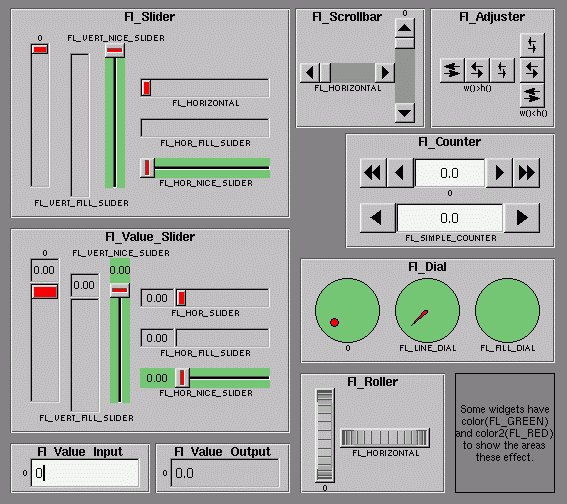# class Fl_Valuator

## Name

class Fl_Valuator -- floating-point value widget

## Class Hierarchy

```Fl_Widget
|
+----Fl_Valuator
|
Fl_Slider, Fl_Value_Input, Fl_Value_Output,```

## Include Files

`#include <FL/Fl_Valuator.H>`

## Description

The Fl_Valuator class controls a single floating-point value and provides a consistent interface to set the value, range, and step, and insures that callbacks are done the same for every object.

There are probably more of these classes in FLTK than any others:In the above diagram each box surrounds an actual subclass. These are further differentiated by setting the type() of the widget to the symbolic value labeling the widget. The ones labelled "0" are the default versions with a type(0). For consistency the symbol FL_VERTICAL is defined as zero.

## Methods

`Fl_Valuator::Fl_Valuator(int x, int y, int w, int h, const char *label = 0)`

Creates a new Fl_Valuator widget using the given position, size, and label string. The default boxtype is FL_NO_BOX.

`virtual Fl_Valuator::~Fl_Valuator()`

Destroys the valuator.

```double Fl_Valuator::value() const
int Fl_Valuator::value(double)```

Get or set the current value. The new value is not clamped or otherwise changed before storing it. Use clamp() or round() to modify the value before calling this if you want. If the new value is different than the current one the object is redrawn. The initial value is zero.

```double Fl_Valuator::minimum() const
void Fl_Valuator::minimum(double)```

Gets or sets the minimum value for the valuator.

```double Fl_Valuator::maximum() const
void Fl_Valuator::maximum(double)```

Gets or sets the maximum value for the valuator.

`void Fl_Valuator::range(double min, double max);`

Sets the minimum and maximum values for the valuator. When the user manipulates the widget, the value is limited to this range. This clamping is done after rounding to the step value (this makes a difference if the range is not a multiple of the step).

The minimum may be greater than the maximum. This has the effect of "reversing" the object so the larger values are in the opposite direction. This also switches which end of the filled sliders is filled.

Some widgets consider this a "soft" range. This means they will stop at the range, but if the user releases and grabs the control again and tries to move it further, it is allowed.

The range may affect the display. You must redraw() the widget after changing the range.

```double Fl_Valuator::step() const
void Fl_Valuator::step(double)
void Fl_Valuator::step(int A, int B)```

Get or set the step value. As the user moves the mouse the value is rounded to the nearest multiple of the step value. This is done before clamping it to the range. For most objects the default step is zero.

For precision the step is stored as the ratio of two integers, A/B. You can set these integers directly. Currently setting a floating point value sets the nearest A/1 or 1/B value possible.

`int Fl_Valuator::format(char *)`

Format the passed value to show enough digits so that for the current step value. If the step has been set to zero then it does a %g format. The characters are written into the passed buffer.

`double Fl_Valuator::round(double)`

Round the passed value to the nearest step increment. Does nothing if step is zero.

`double Fl_Valuator::clamp(double)`

Clamp the passed value to the valuator range.

`double Fl_Valuator::increment(double,int n)`

Adds n times the step value to the passed value. If step was set to zero it uses fabs(maximum() - minimum()) / 100.

`int Fl_Widget::changed() const`

This value is true if the user has moved the slider. It is turned off by value(x) and just before doing a callback (the callback can turn it back on if desired).

`void Fl_Widget::set_changed()`

Sets the changed() flag.

`void Fl_Widget::clear_changed()`

Clears the changed() flag.